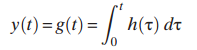### Create an Account

Home / Questions / Use the convolution integral to show that if the input to a linear circuit is x t u t the...

# Use the convolution integral to show that if the input to a linear circuit is x t u t then That is show that the step response is the integral of the impulse response

Use the convolution integral to show that if the input to a linear circuit is x (t) = u (t), thenThat is, show that the step response is the integral of the impulse response.

May 24 2020 View more View LessSubscribe To Get Solution